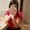## Identifying Percent Amount and Base

• 0:00 - 0:08
We are asked to identify the percent amount and base in this problem.
• 0:08 - 0:12
They ask us 150 is 25% of what number?
• 0:12 - 0:20
So another way to think about it is 25% times some number, so I will do 25% in yellow.
• 0:20 - 0:35
And 25% times some number is equal to 150.
• 0:35 - 0:40
So the percent is pretty easy to spot. We have a 25% right over here.
• 0:40 - 0:45
So, this is going to be the percent. That is the percent.
• 0:45 - 0:50
And we are multiplying the percent times some base number. So this right over here is the base
• 0:50 - 0:57
and we have percent times the base is equal to some amount.
• 0:57 - 1:06
• 1:06 - 1:11
This is essentially saying 25% times some number is equal to 150.
• 1:11 - 1:19
If it helps, we can rewrite this as 0.25 (which is the same thing as 25%)
• 1:19 - 1:27
0.25 times some number is equal to 150.
• 1:27 - 1:32
And one interesting thing to think about is "should this number be larger or smaller than 150"?
• 1:32 - 1:35
Well, if we only take 25% of that number, if we only take 25/100 of that number
• 1:35 - 1:46
If we only take 1/4th of that number, because that's what 25% is, we get 150. So this number needs to be larger than 150.
• 1:46 - 1:50
If fact, it has to be larger than 150 by 4.
• 1:50 - 1:52
And to actually figure out what this number is
• 1:52 - 1:57
we can actually multiply, since what is on the left hand side is equal to what is on the right
• 1:57 - 2:01
hand side. If we want to solve this, we can multiply both sides by 4.
• 2:01 - 2:05
If we say, look, we have some value over here and we're going to multiply
• 2:05 - 2:08
it by 4 in order for it to still be equal we would have to multiply 150
• 2:08 - 2:09
times 4.
• 2:09 - 2:16
4 times 0.25 (or 4 times 25% or times 1/4th), this is just going to be 1.
• 2:16 - 2:21
And we are going to get our number is equal to 150 times 4.
• 2:21 - 2:24
Or equal to 600.
• 2:24 - 2:28
And that makes sense. 25% of 600 is 150.
• 2:28 -
1/4th of 600 is 150.
Title:
Identifying Percent Amount and Base
Description:

u5_l2_t1_we1 Identifying Percent Amount and Base

more » « less
Video Language:
English
Duration:
02:32Melanie Karow edited английски език subtitles for Identifying Percent Amount and BaseMelanie Karow added a translation

# English subtitles

## Revisions Compare revisions

• Melanie Karow
• Melanie Karow